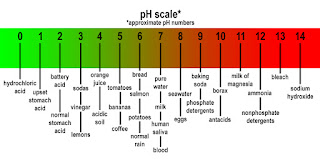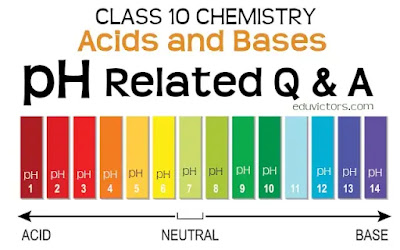# pH related Questions and Answers

CBSE Class 10 - Chemistry

Chapter: Acids and Bases

Q1: How is the strength of acid and base can be estimated?

Answer: Strength of acid and base can be estimated by using universal indicator.

Q2: What is a universal indicator?

Answer: Universal indicator is used to estimate the strength of an acid or a base. It is a mixture of several indicators. It shows different colours at different concentrations of H⁺ ions in the solution.

Q3: What is pH value of a solution?

Answer: The pH of a solution is inversely proportional to the concentration of hydrogen ions in it. Mathematically the pH of a solution is inversely proportional to the concentration of hydrogen ions in it. pH is a pure number, it has no units.

Q4: What is a pH scale?

Answer: A scale for measuring H⁺ ion concentration in a solution . p in pH stands for ‘potenz’ a German word which means power.

The pH scale has values from 0 to 14

pH =7 — neutral solution

pH less than 7 — acidic solution

pH more than 7 — basic solution

Acids have higher concentrations of H+ ions compared to OH- ions. An acid that has a high concentration of H+ ions will have a lower pH value than an acid with a low concentration of H+ ions.

Bases have higher concentrations of OH- ions compared to H+ ions. A base that has a high concentration of OH- ions will have a higher pH value than a base with a low concentration of OH- ions.

Q5: Name the scientist who devised pH Scale.pH Scale of Liquids (credits: pixy.org)

Q6: What is the pH range of the following substances?

(a) Gastric juice

(b) Vinegar

(c) Tears

(d) Human urine

(e) Blood plasma

(f) Boiled water

(g) Battery Water

(h) Tap Water / Drinking Water

(a) Gastric juice = 1-3

(b) Vinegar = 2.4 - 3.4

(c) Tears = 7.4

(d) Human urine = 4.8- 8.4

(e) Blood plasma = 7.3 - 7.4

(f) Boiled water = 6.5

(g) Battery Water = 7.0

(h) Tap Water/ Drinking Water  = 6.5 - 8.5

Q7: What is the pH of a neutral solution?

Q8: Which is more acidic : a solution of pH = 2 or a solution of pH = 6 ?

Answer: Solution of pH = 2 is more acidic.

Q9: Why does tooth decay start when the pH of mouth is lower than 5.5?

Answer: Tooth decay start when the pH of mouth is lower than 5.5 because the acid becomes strong enough to attack the enamel of the teeth and corrode it.

Q10: Which is more basic (or more alkaline) : a solution of pH = 8 or a solution of pH = 11 ?

Answer: Solution of pH = 11

Q11: The pH of soil A is 7.5 while that of soil B is 4.5. Which of the two soils, A or B, should be treated with powdered chalk to adjust its pH and why ?

Answer: Powdered chalk is limestone (CaCO₃) is a basic salt. Soil B is acidic in nature so its treated with powdered chalk to reduce its acidity.

Q12: What colour will universal indicator show if you add it to the following substances ?

(a) potassium hydroxide, pH = 12

(b) soda water, pH = 5

(c) sulphuric acid, pH = 2

(a) Dark Purple

(b) Orange Yellow

(c) Red

Q13: What is the pH of pure water? What is its effect on litmus paper or on methyl orange.

Answer: Pure water is neutral. Its pH is 7. It wil have no effect on litmus or methyl orange.

Q14: On diluting an acid, will its pH increase or decrease?

Answer: On diluting an acid, its pH value increases.

Q15: What is liming of soil?

Answer: Plants are sensitive to changes in soil pH. The pH levels can be controlled by adding certain chemicals. For acidic soil, bases such as calcium oxide (quicklime) and calcium hydroxide (slaked lime) can be added to neutralise the excess H⁺ ions. This process is known as ‘liming’.

Excess of liming increasses the alkalinity of the soil, which can also be harmful to plants.

Q16: Fresh milk has a pH of 6. When it changes into curd (yogurt), will its pH value increase or decrease? Why?

Answer: pH value will decrease when milk changes to curd. Curd contains lactic acid hence the pH decreases.

Q17: How does the concentration of Hydronium ions (H₃O⁺) affected when a solution of an acid is diluted?

Answer: The concentration of Hydronium ions (H₃O⁺) per unit volume decreases when a solution of acid is diluted. Hence its pH value increases.

Q18: Name the acid present in bee sting?

Answer: Formic acid or Methanoic acid.

Q19: Explain how pH in our digestive system is maintained?

1.  Our stomach produces HCl acid which helps in digestion. The acid kills harm germs present in the ingested food.

2.  During indigestion, stomach produces more acid and cause pain and irritation.

3. To get rid of this pain, people uses antacid (mild base) like milk of magnesia [Mg(OH)₂] to neutralize excess acid.

4. The acidic food enters in small intestine, the bile juice (basic in nature) from liver neutralises the acidic medium.

Q20: Explain how pH change in the lake water can endanger the lives of aquatic animals (like fish). What can be done to lessen the danger to the lives of aquatic animals in the lake?

Answer: The pH of lake water becomes lower because of too much acid rain. The high acidity of lake water can kill the aquatic animals like fish since they can survive within a narrow range of pH change. Calcium carbonate is added to acidic lake water to neutralise the acid and this prevents the fish from being killed.

Q21: What colour would the universal indicator show in an aqueous solution of sugar ? Why ?

Answer: Aqueous solution of sugar will turn the color of universal indicator green because sugar solution is neutral in nature.

Q22: A sample of rain water turned universal indicator paper yellow. What would you expect its pH to be ? Is it a strong or a weak acid ?

Answer: pH of the sample of rain water will be between 5 and 6. It is a weak acid. Rain water with ph value less than 5.5 is called acid rain.

Q23: What do you think will be the pH of an antacid solution : less than 7 or more than 7 ?

Answer: Antacids are a group of mild bases so they have pH more than 7.

Q24: Name two common antacids.

Answer: Antacids: Magnesium hydroxide and Sodium hydrogencarbonate.

Q25: Do basic solutions also have H (aq) ions ? If yes, then why are they basic ?

Answer: Yes, all basic solutions have H ions. They are basic because the concentration of hydrogen ions is much less than that of hydroxide ions.